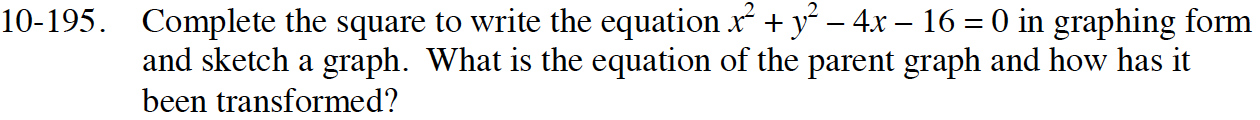### Home > INT3 > Chapter Ch10 > Lesson 10.3.3 > Problem10-195

10-195.

Complete the square to write the equation x2 + y2 – 4x – 16 = 0 in graphing form and sketch a graph. What is the equation of the parent graph and how has it been transformed? Homework Help ✎Gather the x terms together andmove the constants to the right side of the equation.

(x2 − 4x + ?) + y2 = 16

Determine what value will complete the square and add it to both sides of the equation.

(x2 − 4x + 4) + y2 = 16 + 4

Factor and identify the center of the circle and the radius.

(x − 2)2 + y2 = 20